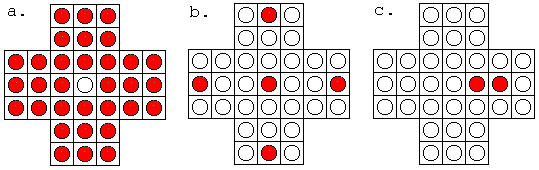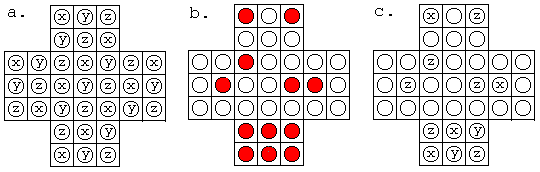# Peg Solitaire and Group Theory

Peg Solitaire (also known as Hi-Q) has very simple rules. Pegs (red circles) are allowed to jump over adjacent (vertically or horizontally) pegs. The peg that has been jumped over is removed. So jumps are like captures in Checkers.

The goal of a regular game is to remove all pegs but one. In central solitaire, the player starts with pegs filling all the holes, except for the central one. According to the game brochure (Milton Bradley Co., 1986), whoever succeeds in leaving the last peg in the center is a genius. Anyone who leaves a single peg elsewhere is an outstanding player.Figure 1

Not long ago, with the help of very elementary group theory, Arie Bialostocki from University of Idaho proved that there are only five locations (b. above) where one can leave that single peg. Assuming that, e.g., the peg was left in the rightmost hole, part c. in Figure 1 shows the position before the last move. The irony is in that from the same position the player can leave the sole remaining peg in the central hole, thus gaining the status of genius, instead of an outstanding player. Would one trade the distinction? It's this amazing observation that led Arie Bialostocki to developing his nice theory which I am going to outline below.Figure 2

Place letters x, y, z as shown in Figure 2a. The arrangement of letters is very special and has been noticed yet in the classic WW, page 706. Whenever one of the letters points to a peg that jumps over a peg with another letter on it it always lands in a hole labeled by the third letter.

Moves Over To x y z x z y y x z y z x z x y z y x

We may define an operation "+" on letters x, y, z to shorten move Description. Let's write x + y = z to indicate the fact expressed in the first row of the table, namely, that whenever peg x jumps over peg y it always lands in hole z. Similar notion are used for the remaining rows of the table, so that, for example, y + x = z and z + x = y and so on.

The operation thus defined is commutative. Indeed, for example, z + x = y, but also x + z = y, so that z + x = x + z. Further, the operation "+" has been defined for all pairs of three letters, other than x + x, y + y, and z + z. Guided by Group theory, we may set

x + x = y + y = z + z = 0,

where the new symbol 0 is required to fulfill

x + 0 = x, y + 0 = y and z + 0 = z.

All the properties of the operation "+" can now be summarized in its Cayley table:

0 x y z 0 x y z x 0 z y y z 0 x z y x 0

The Cayley table of a group collects all the information about the group operation ("+" in our case) in compact form.

An additional property of "+" can be derived now from its Cayley table, namely, the sum of all three non-zero symbols x, y, z in any order is always 0: x + y + z = 0. (Does not this remind you of 3-purges?) We can also consider the sum of all pegs in a configuration (See Figure 2b-c.) For example, it is clear that the sum of all pegs in the starting position of central solitaire is y - the value of the sole unoccupied hole. When x jumps over y it lands in z. So that two pegs x + y are replaced with a single peg z, which means that the moves in peg solitaire do not change the value of the game's configuration. In other words, the value of a position in peg solitaire is invariant under the legal moves. In particular,

Any position derived in central solitaire by legal moves has the value of y!

This is of course true of any position with a single peg left.Figure 3

Therefore, the only possible locations for the sole remaining peg are those indicated in Figure 3a. However, not all locations are attainable. Since the starting configuration has both central and line symmetry, and since moves may also follow any of those symmetries, if, for example, a single peg might be left in the "corner" position in Figure 3b, it would be also possible to leave a single peg in other "corner" positions, like that in Figure 3c. But position in Figure 3c is labeled by z. Therefore, the position in Figure 3b is not possible. The only positions that withstand the symmetry test are the five in Figure 1b.A. Bialostocki mentions in a personal introduction to the paper that his Erdös number is 1. As I have recently learned, mine is 3. The reason is that I once wrote a paper with Ken Atkinson from University of Iowa whose Erdös number is 2, which means that he wrote a paper with somebody whose Erdös number is 1, which in turn means that the latter is one of a host of Erdös' co-authors.

## Reference

1. K. Atkinson and A. Bogomolny, The discrete Galerkin method for integral equations, Math of Comp 48 (1987),595-616 and S11-S15.
2. E. R. Berlekamp, J. H. Conway, R. K. Guy, Winning Ways for Your Mathematical Plays, v2, Academic Press, 1982.
3. A. Bialostocki, An Application of Elementary Group Theory to Central Solitaire, The College Mathematics Journal, v 29, n 3, May 1998, 208-212.### What Can Be Multiplied?•